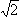#Interactive Real Analysis

Next | Previous | Glossary | Map

## 2.4. The Real Number System

### Examples 2.4.3(b):

Consider the set of rational numbers {1, 1.4, 1.41, 1.414, 1.4142, ...} converging to the square root of 2. If all we knew were rational numbers, this set would have no supremum. If we allow real numbers, there is a unique supremem.
If we consider the universe to consist only of rational numbers, then this set does not have a least upper bound.
• No number bigger thanis the least upper bound (although each of these numbers is an upper bound), because if x was that least upper bound, then we can find a rational number betweenand x. That rational number would then be an upper bound smaller than x, which is a contradiction.
• No number less thanis the least upper bound, because if x was that least upper bound, there is some element of the set between x and. But then x is not an upper bound, which is a contradiction.
Hence, there is no supremum for this set S in Q.

If we consider this set as a subset of the real numbers, then the least upper bound of this set is.

Next | Previous | Glossary | Map